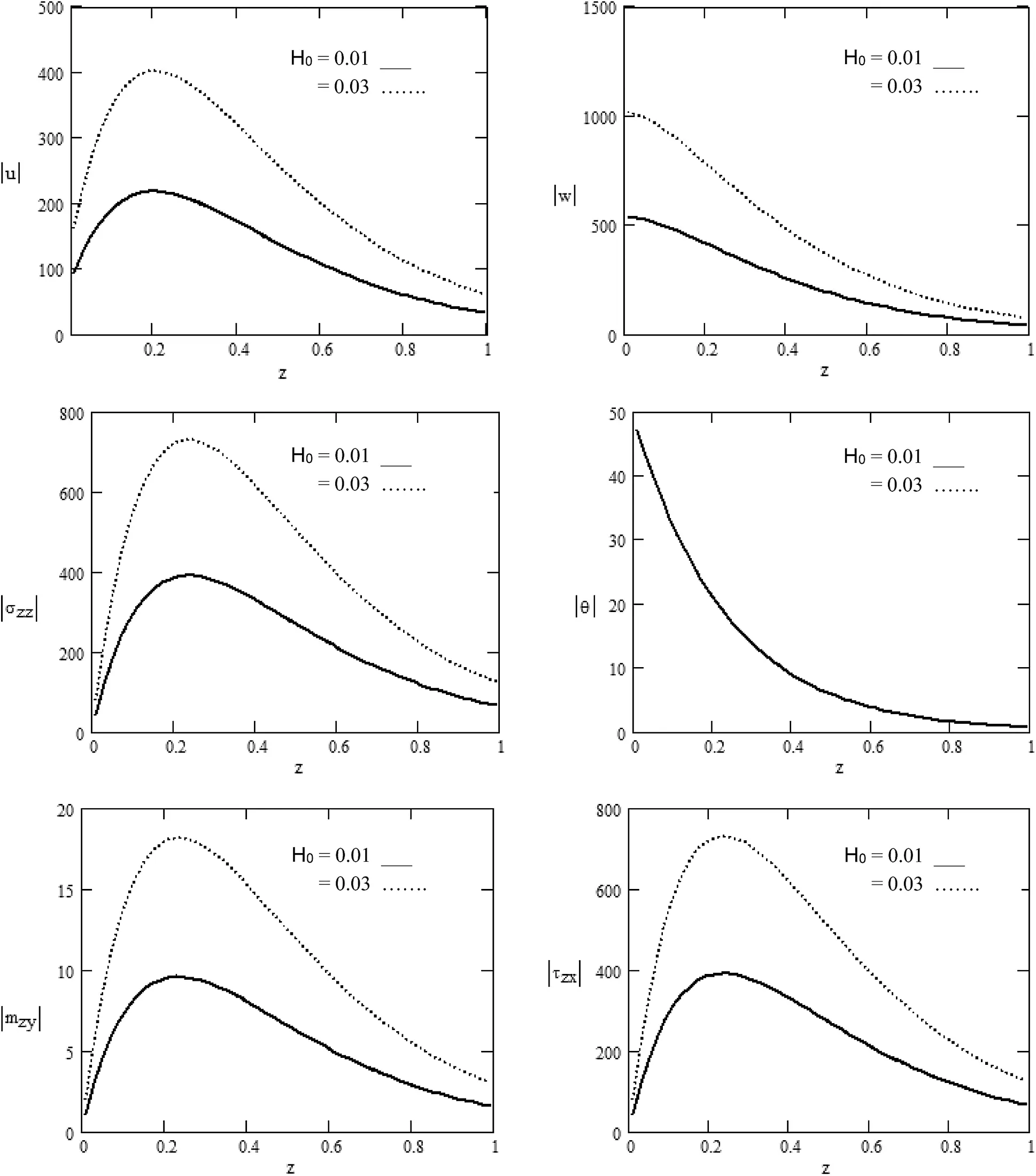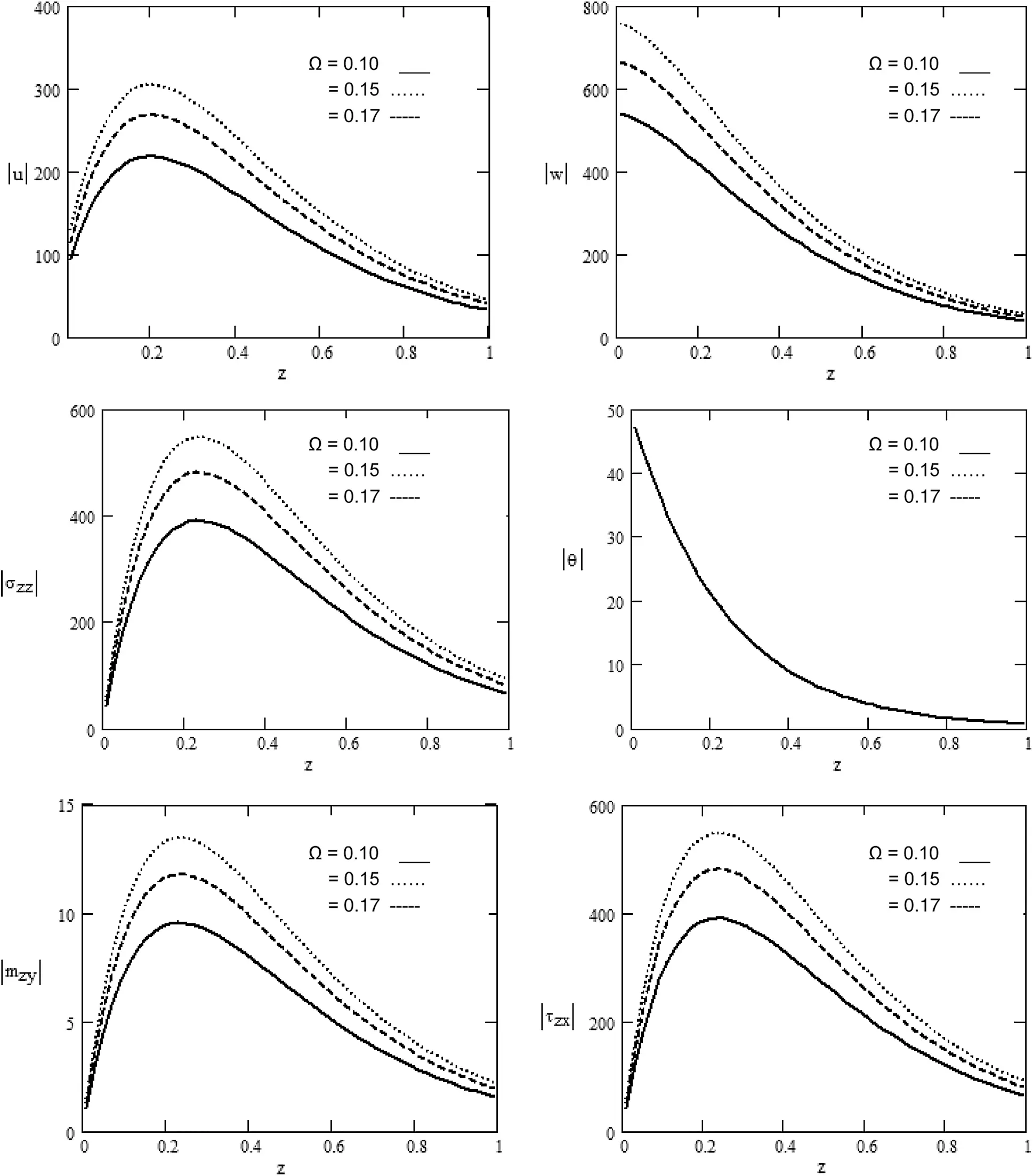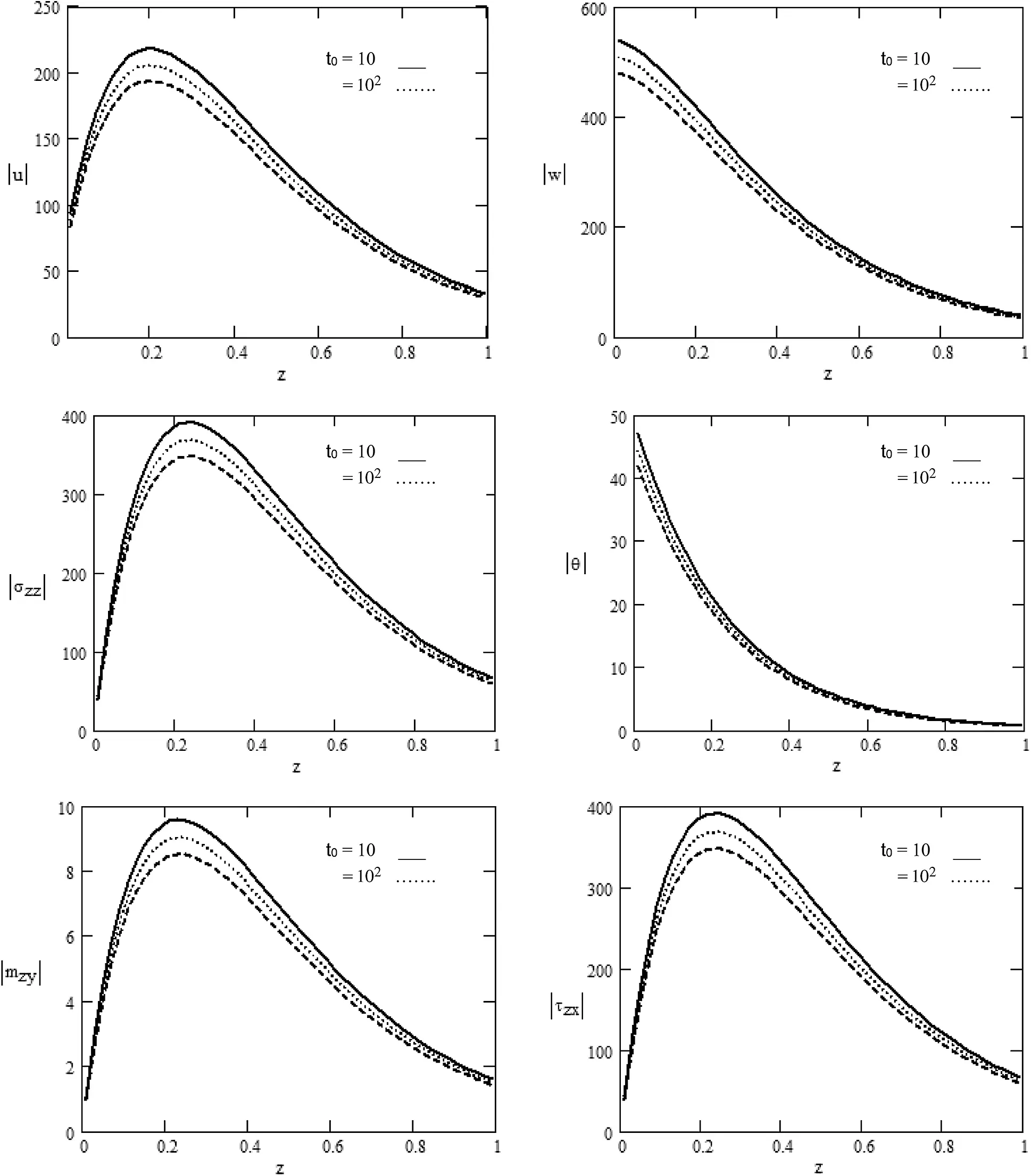[BACK]
 Computers, Materials & ContinuaDOI:10.32604/cmc.2021.015563Article

Rotational Effect on the Propagation of Waves in a Magneto-Micropolar Thermoelastic Medium

1Department of Mathematics, Faculty of Science, Sohag University, Sohag, Egypt
2Department of Mathematics, Faculty of Science, South Valley University, 83523, Egypt
3Department of Physics, Faculty of Science, Jazan University, Jazan, Saudi Arabia
4Department of Physics, Faculty of Science, Zagazig University, Zagazig, Egypt
*Corresponding Author: A. M. Abd-Alla. Email: mohmrram@yahoo.com
Received: 28 November 2020; Accepted: 14 February 2021

Abstract: The present paper aims to explore how the magnetic field, ramp parameter, and rotation affect a generalized micropolar thermoelastic medium that is standardized isotropic within the half-space. By employing normal mode analysis and Lame’s potential theory, the authors could express analytically the components of displacement, stress, couple stress, and temperature field in the physical domain. They calculated such manners of expression numerically and plotted the matching graphs to highlight and make comparisons with theoretical findings. The highlights of the paper cover the impacts of various parameters on the rotating micropolar thermoelastic half-space. Nevertheless, the non-dimensional temperature is not affected by the rotation and the magnetic field. Specific attention is paid to studying the impact of the magnetic field, rotation, and ramp parameter of the distribution of temperature, displacement, stress, and couple stress. The study highlighted the significant impact of the rotation, magnetic field, and ramp parameter on the micropolar thermoelastic medium. In conclusion, graphical presentations were provided to evaluate the impacts of different parameters on the propagation of plane waves in thermoelastic media of different nature. The study may help the designers and engineers develop a structural control system in several applied fields.

Keywords: Magnetic field; micropolar; thermoelastic; rotation; ramp parameter; normal mode analysis

Nomenclature

 σij: Stress tensor components mij: Couple stress tensor components ϕ: Micro–rotation λ,μ: Lame’s constants α,β,γ,k: Micropolar material constants u→: Components of the displacement vector β1=αt(3λ+2μ+k) αt: Linear thermal expansion coefficient ρ: Density of the medium e: Cubical dilatation K*: Thermal conductivity K1*=cE(λ+2μ)4 cE: Specific heat at constant strain Ω: Angular temperature θ=T-T0 T0: The medium’s temperature in its material state supposed to be |θT0|<<1

1  Introduction

Because it is relevant in several applications, researchers have paid due attention to generalized thermoelasticity. Its theories include governing equations of the hyperbolic type and disclose the thermal signals’ finite speed. In a standardized thermoelastic isotropic half-space, Abd-Alla et al.  investigated how wave propagation is affected by a magnetic field. Abouelregal  discussed the improved fractional photo-thermoelastic system for a magnetic field- subjected rotating semiconductor half-space. Singh et al.  studied reflecting plane waves from a solid thermoelastic micropolar half-space with impedance boundary circumstances. Said  studied the propagation of waves in a two-temperature micropolar magnetothermoelastic medium for a three-phase-lag system. The authors of  explored the impact of rotation on a two-temperature micropolar standardized thermoelasticity utilizing a dual-phase lag system. Rupender  studied the impact of rotation on a micropolar magnetothermoelastic medium because of thermal and mechanical sources. Bayones et al.  investigated the impact of the magnetic field and rotation on free vibrations within a non-standardized spherical within an elastic medium. Bayones et al.  discussed the thermoelastic wave propagation within the half-space of an isotropic standardized material affected by initial stress and rotation. Kalkal et al.  investigated reflecting plane waves in a thermoelastic micropolar nonlocal medium affected by rotation. Lotfy  explored two-temperature standardized magneto-thermoelastic responses within an elastic medium under three models. Zakaria  discussed the effect of hall current on a standardized magneto-thermoelasticity micropolar solid under heating of the ramp kind. Ezzata et al.  studied the impacts of the fractional order of heat transfer and heat conduction on a substantially long hollow cylinder that conducts perfectly. Morse et al.  employed Helmholtz’s theorem. The authors of  investigated the transient magneto-thermoelasto-diffusive interactions of the rotating media with porous in the absence of the dissipation of energy influenced by thermal shock. Kumar et al.  studied the axisymmetric issue within a thermoelastic micropolar standardized half-space. The authors of  studied the free surface’s reflection of the thermoelastic rotating medium reinforced with fibers with the phase-lag and two temperatures. Deswal et al.  studied the law of fractional-order thermal conductivity within a two-temperature micropolar thermo-viscoelastic. Using radial ribs, the authors of  examined the improved rigidity of fused circular plates. The authors of  investigated the motion equation for a flexible element with one dimension utilized for the dynamical analysis of a multimedia structure.

The paper aims to study the thermoelastic interactions within an elastic standardized micropolar isotropic medium in the presence of rotation affected by a magnetic field. The authors could obtain accurate solutions of the measured factors using the Lame’s potential theory and the normal mode analysis. They could obtain and present in graphs the numerical findings of the distributions of stress, displacement, and temperature, for a crystal-like magnesium material.

2  Constitutive Relations and Field Equations

Constitutive relations and field equations are considered within a generalized micropolar magneto-thermoelastic medium in the presence of rotation as:

(i) The Constitutive Relations

σij=λur,rδij+μ(ui,j+uj,i)+k(uj,i-εijrϕr)-β1θδij,(1)

mij=αϕr,rδij+βϕi,j+γϕj,i(2)

(ii) Motion’s Stress Equation

(λ+μ)(.u)+(μ+k)2u+k(xϕ)-β1θ+f=ρ(2ut2)+Ωx(Ωxu)+2Ωxu.(3)

(iii) Motion’s Couple Stress Equation

(α+β+γ)(.ϕ)-γx(xϕ)+kxu-2kϕ=ρJ2ϕt2.(4)

(iv) The Equation of Thermal Conductivity

K1*(1+K*t)2θ=ρcE(θ¨+β1T0e¨(5)

For these equations, the authors use the summation convention and utilize the comma to signify material derivative.

3  Formulating the Problem

Take into account an isotropic standardized micropolar generalized magneto-thermoelastic in the presence of rotation. The rectangular Cartesian coordinate scheme (x,y,z) is used in which the half-space surface plays the role of the plane z = 0 and the zaxis points vertically inwards. There are two extra terms for the displacement motion equation within the rotating frame, i.e., the Coriolis acceleration 2Ωxu̇ because of the moving frame of reference and the centripetal acceleration Ωx(Ωxu) because of the motion that varies over time. The analysis is restricted to the xz plane. Therefore, the quantities within the medium do not depend on the variable y. The angular velocity Ω, displacement vector u, magnetic field vector H and micro-rotation vector ϕ have the components

u=u(x,z,t),w=w(x,z,t),Ω=(0,Ω,0),H=(0,H0,0),ϕ=(0,ϕ2,0).(5a)

J=curlh,(6a)

curlE=-μeht,(6b)

divh=0,(6c)

divE=0,(6d)

E=-μe(ut×H),(6e)

h=curl(uxH).(6f)

in which u represents the displacement vector, H represents the magnetic field, μe represents the magnetic permeability, J represents the density of the electric current, E represents the electric intensity, and h represents the perturbed magnetic field over the principal magnetic field. We apply the initial magnetic field vector H in Cartesian coordinates (x,y,z) to Eq. (1)

u=(u,0,w),H=(0,H0,0),E=-μe(-H0wt,0,H0ut),h=(-H0w,0,H0u),j=-H0(ux+wz),f=μe(jxH).(7)

where f is the Lorentz force.

Combining Eqs. (3), (4) and Eqs. (5a), (7) provides

(λ+μ)x(ux+wz)+(μ+k)2u-kϕ2z-β1θx+μeH02(2ux2+2wxz)=ρ(2ut2-Ω2u+2Ωwt),(8)

(λ+μ)z(ux+wz)+(μ+k)2w-kϕ2x+β1θz+μeH02(2wz2+2uxz)=ρ(2wt2-Ω2w-2Ωut),(9)

γ2ϕ2+k(uz-wx)-2kϕ2=ρj2ϕ2t2.(10)

The following form expresses Eq. (5) of thermal conductivity given expression (5a)

(K1*(1+K*t)2θ=2t2(ρcEθ+β1*T0e).(11)

Substituting (5a) into (1) and (2), we can express the arising stress as

σxx=(λ+2μ+k)ux+λwz-β1θ,(12)

σzz=(λ+2μ+k)wz+λux-β1θ,(13)

τzx=μwx+(μ+k)uz-kϕ2,(14)

mzy=γϕ2z,mxy=γϕ2x.(15)

where

e=ux+wz,2=2x2+2z2,

These variables that are non-dimensional are employed to change the previous equations into non-dimensional structures

(x,z)=ω*c1(x,z),t=ω*t,(u,w)=ρω*c1T0β1(u,w),θ=θT0,Ω=Ωω*,σij=σijT0β1,ϕ2=ρc12T0β1ϕ2,mij=ω*c1T0β1mij.(16)

where

ω*=ρc12cEK*,c12=(λ+2μ+k)ρ,β1=(3λ+2μ+k)αt.

Eqs. (8)(11) concerning previous the variables that are non-dimensional decline to (dropping the primes)

(λ+μ)x(ux+wz)+(μ+k)2u-kϕ2z-β1ρc12β1eθx+μeH02(2ux2+2wxz)=ρc12(2ut2-Ω2u+2Ωwt),(17)

(λ+μ)z(ux+wz)+(μ+k)2w+kϕ2z-β1ρc12β1eθz+μeH02(2wz2+2uxz)=ρc12(2wt2-Ω2w-2Ωut),(18)

γc122ϕ2+kω*2(uz-wx-2ϕ2)=ρj2ϕ2t2,(19)

[K1*+K*ω*t]ω*2T0c122θ=ω*22t2(ρcET0θ+β1*T02β1eρc12e(20)

Utilizing Helmholtz decomposition  and providing the potential ϕ and ψ through the following equation

u=ϕ+ψ,ψ=(0,-ψ,0).(21)

Using Eq. (5a), the components of displacement u and w take the following form

u=ϕx+ψz,w=ϕz-ψx.(22)

where

ϕ(x,z,t) and ψ(x,z,t) represent scalar potential functions and ψ represents the vector of the potential function

Substituting Eq. (22) into Eqs. (17)(20) gives us

(2-2t2+Ω2)ϕ-(1+β1t)θ+2Ωψt=0,(23)

(a0+a2+4t)ψ-2Ωϕt-a0ϕ2=0,(24)

(2-2a3-a42t2)ϕ2+a32ψ=0,(25)

({(a1+2a2)+4t}-42t2)θ-4a52t22ϕ=0.(26)

where

a0=kρc12,a1=λρc12,a2=μρc12,a3=kc12γω*2,a4=jρc12γ,a5=T0β12ρK*ω*.

4  Normal Mode Analysis

For resolving the governing equations, the authors measured physically the decomposed variable concerning the normal modes in the following form:

(u,w,ϕ,ψ,θ,σij,ϕ2)(x,z,t)=(u*,w*,ϕ*,ψ*,θ*,σij*,ϕ2*)(z)e(ωt+imx).(27)

in which ω represents the complex time constant (frequency), i represents the imaginary unit, m represents the wave number in the x-direction, and u*,w*,ϕ*,ψ*,θ*,σij* and ϕ2* represent the functions’ amplitudes.

Using (27), Eqs. (23)(25) and Eq. (20) are, as follows

(D2-A1)ϕ*(z)-A2θ*(z)+A3ψ*(z)=0,(28)

(D2-B1)ψ*(z)-B2ϕ*(z)-B3ϕ2*(z)=0,(29)

(D2-C1)ϕ2*(z)+a3(D2-m2)ψ*(z)=0,(30)

(D1(D2-m2)-D3)θ*(z)-D2(D2-m2)ϕ*(z)=0.(31)

in which

A1=m2+ω2-Ω2ε1,A2=1+β1ωε1,A3=2Ωωε1,B1=m2+ω2-Ω2ε2,B2=2Ωωε2,B3=a0ε2,C1=m2+2a3+a4ω2,D1=(a1+2a2)(1+τvω)+4ω(1+τTω),D2=4a5ω2,D3=4ω2,ε1=1+μeH02ρc12,ε2=a0+a2.

Eliminating ψ*(z),ϕ*(z),ϕ2*(z) and θ*(z) from Eqs. (28)(31), the differential equation become

(D8-PD6+QD4-RD2+S)(ψ*(z),ϕ*(z),ϕ2*(z),θ*(z))=0.(32)

in which

P=A1+B1+C1+m2+ε3(D3+A2D2)-a3B3,Q=A1B1+A1C1+B1C1+(m2+ε3D3)(A1+B1+C1)+ε3A2D2(B1+C1+m2)+A3B2-a3B3(2m2+A1+ε3(D3+A2D2)),R=A1B1C1+(m2+ε3D3)(A1B1+B1C1+A1C1)+ε3A2D2(m2(B1+C1)+B1C1)+A3B2(m2+C1+ε3D3)-a3B3(m2A1+(m2+A1)(m2+ε3D3)+2ε3m2A2D2),S=(m2+ε3D3)(A1B1C1+A3B2C1)+ε3m2A2D2B1C1-a3B3(m2A1(m2+ε3D3)+ε3m4A2D2).

in which ε3=1D1.

Eq. (27) is represented, as follows

((D2-λ12)(D2-λ22)(D2-λ32)(D2-λ42))(ψ*(z),ϕ*(z),ϕ2*(z),θ*(z))=0.(33)

in which λn2 (n = 1, 2, 3, 4) represent the roots of the characteristic Eq. (32).

λ1=123((3e1-6e2)),λ2=123((3e1+6e2)),λ3=123((3e1-6e2)),λ4=123((3e1+6e2)).(34)

where

Θ=2Q3-9PQR+27R2+27P2S-72QS,Θ1=Q2-3PR+12S,Θ2=3Q3-9Q(PR+8S)+27(R2+P2S),Θ3=(Θ+-Θ1 3+Θ22)13,Θ4=43(P3-4PQ+8R),e1=(3P28Q-273Θ1Θ3+253Θ3),e2=(3P2-8Q-243Θ1Θ3-223Θ3-e3),e3=Θ4e1.

The solution of Eq. (28) is written as

ψ*(z)=n=14Mn(m,ω)e-λnz,(35)

ϕ*(z)=n=14Mn(m,ω)e-λnz,(36)

ϕ2*(z)=n=14Mn(m,ω)e-λnz,(37)

θ*(z)=n=14Mn(m,ω)e-λnz.(38)

in which Mn,Mn,Mn and M″′n represent some parameters that depend on m and ω. Substitution of Eqs. (35)(38) into Eqs. (28)(31) gives the following relations

ϕ2*(z)=n=14S1nMn(m,ω)e-λnz,(39)

ϕ*(z)=n=14S2nMn(m,ω)e-λnz,(40)

θ*(z)=n=14S3nMn(m,ω)e-λnz.(41)

where

S1n=-a3(λn2-m2)(λn2-C1),S2n=(λn2-B1)B2-B3B2S1n,S3n=D3(λn2-m2)S2nD1(λn2-m2)-D3.

5  Applications

Take into account a magneto-thermoelastic micropolar solid having a half-space that rotates z0. Mns, as constants, are defined by imposing the proper boundary settings.

5.1 Thermal Boundary Conditions

The authors apply a heat shock of the ramp kind to the isothermal boundary of the plane z = 0, as follows

θ(x,0,t)=ϕ*δ(x)H(t).(42)

in which δ(x) represents Dirac-delta function and ϕ* represents a constant temperature. Moreover, H(t) represents a Heaviside function defined as

H(t)={0t0tt00tt01tt0(43)

In this equation, t0 represents a ramp parameter that shows the time required for raising the heat.

5.2 Mechanical Boundary Conditions

The boundary plane z = 0 is free of traction. In terms of mathematics, the boundary conditions take the following form

σzz(x,0,t)=0,(44)

τzx(x,0,t)=0,(45)

mzy(x,0,t)=0.(46)

Applying Eqs. (16)(27) make the former boundary conditions represented, as follows

θ*(z)=R1,(47)

σzz*(z)=0,(48)

τzx*(z)=0,(49)

mzy*(z)=0.atz=0(50)

where R1=ϕ*1-e-ω0t0ω2.

Utilizing the non-dimensional quantities identified in (16), expressing stress Eqs. (12)(15) and displacement Eq. (22), as well as the relations (44)(46) becomes:

u*(z)=n=14(imS2n-λn)Mn(m,ω)e-λnz,(51)

w*(z)=n=14(-λn-im)Mn(m,ω)e-λnz,(52)

σzz*(z)=n=14S4nMn(m,ω)e-λnz,(53)

τzx*(z)=n=14S5nMn(m,ω)e-λnz,(54)

mzy*(z)=n=14S6nMn(m,ω)e-λnz.(55)

in which

S4n=((a0+a1(1+α0ω)+2a2(1+α1ω))λn2-m2a1(1+α0ω))S2n+imλn(a0+2a2(1+α1ω)-(1+β1ω)S3n,S5n=(a0+a2(1+α1ω))λn2-imλnS2n(a0+2a2(1+α1ω))+m2a2(1+α1ω))-a0S1n,S6n=-a0a3λnS1n.

A four-equation non-homogeneous structure is yielded by the boundary conditions (47)(50), supported by expressions (51)(55) and (38) as follows

[S31S32S33S34S41S42S43S44S51S52S53S54S61S62S63S64][M1M2M3M4]=[R1000](56)

The expressions of Mn, (n = 1, 2, 3, 4) attained by resolving the structure (56), when replaced in Eq. (38) and Eqs. (51)(55), give these expressions of field variables

u(x,z,t)=[(imS21-λ1)M1e-λ1z+(imS22-λ2)M2e-λ2z+(imS23-λ3)M3e-λ3z+(imS24-λ4)M4e-λ4z]e(ωt+imx),(57)

w(x,z,t)=-[(λ1S21+im)M1e-λ1z+(λ2S22+im)M2e-λ2z+(λ3S23+im)M3e-λ3z+(λ4S24+im)M4e-λ4z]e(ωt+imx),(58)

θ(x,z,t)=[S31M1e-λ1z+S32M2e-λ2z+S33M3e-λ3z+S34M4e-λ4z]eωt+imx,(59)

σzz(x,z,t)=[S41M1e-λ1z+S42M2e-λ2z+S43M3e-λ3z+S44M4e-λ4z]eωt+imx,(60)

τzx(x,z,t)=[S51M1e-λ1z+S52M2e-λ2z+S53M3e-λ3z+S54M4e-λ4z]eωt+imx,(61)

mzy(x,z,t)=[S61M1e-λ1z+S62M2e-λ2z+S63M3e-λ3z+S64M4e-λ4z]eωt+imx.(62)

in which

M1=Δ1Δ,M2=Δ2Δ,M3=Δ3Δ,M4=Δ4Δ,Δ=S31d1-S32d2+S33d3-S34d4,Δ1=R1d1,Δ2=-R1d2,Δ3=R1d3,Δ4=-R1d4,d1=S42(S53S64-S54S63)-S43(S52S64-S54S62)+S44(S52S63-S53S62),d2=S41(S53S64-S54S63)-S43(S51S64-S54S61)+S44(S51S63-S53S61),d3=S41(S52S64-S54S62)-S42(S51S64-S54S61)+S44(S51S62-S61S52),d4=S41(S52S63-S53S62)-S42(S51S63-S61S53)+S43(S51S62-S61S52).

6  Numerical Results and Discussion

With an aim to highlight the theoretical findings of the previous sections, the authors provide some numerical findings using MATLAB. The material selected for this goal of magnesium crystal whose physical data resemble those provided in :

ρ=1.74×103kgm-3,λ=9.4×1010kgm-1s-2,μ=4.0×1010kgm-1s-2,k=1.0×1010kgm-1s-2,γ=0.779×10-9kgms-2,j=0.2×10-19m2,αt=2.36×10-5K-1,K*=2.510Wm-1K-1,T0=293K,CE=9.623×102Jkg-1K-1,z=0.3ϕ*=1.0.

Taking into account the previous physical data, non-dimensional field variables are estimated, and the findings are presented graphically at various positions of z at t = 0.1 and x = 1.0. The motion range is 0z1.0. Figs. 13 show variations, correspondingly.Figure 1: Various values of the magnitude of u,w,σzz,τzx,mzy,θ for various values of H0 in the presence of distance 0z1Figure 2: Various values of the magnitude of u,w,σzz,τzx,mzy,θ for various values of Ω in the presence of distance 0z1Figure 3: Various values of the magnitude of u,w,σzz,τzx,mzy,θ for various values of t0 in the presence of distance 0z1

Fig. 1: displays the various values of the components of displacement |u| and |w|, stress |σzz|, |τxz|,|mzy|, as well as temperature |θ| in the presence of distance z for the various values of the magnetic field H0. A zero value is the beginning. It agrees entirely with the boundary conditions. The magnetic field demonstrates significant growing and declining impacts on the components of stress and displacement. These impacts vanish when moving away from the point of the application of the source. The temperature is not affected by the magnetic field. Nevertheless, the qualitative performance is almost equal in the two values.

Variations in displacement components |u|,|w|, stress components |σzz|, |τxz|,|mzy| and temperature |θ| with spatial coordinates z for various values of rotation Ω are shown in Fig. 2 that begins with the value of zero, which agrees totally with the boundary conditions. The highest impact zone of rotation is about z = 0.3. Nevertheless, the temperature is not affected by the rotation. The components of temperature, stress, and displacement increase numerically for 0z1. Then, they decrease and moves to minimum value at z = 1.

In Fig. 3, we have depicted displacement components |u|,|w|, stress components |σzz|, |τxz|,|mzy| and temperature |θ| in the presence of distance z to explore the impacts of ramp parameter t0. The ramp parameter’s highest impact zone is about z = 0.3. Furthermore, all curves share a corresponding zero value stating point to satisfy the boundary conditions.

7  Conclusion

The study concludes that:

a) The magnetic field and rotation play an influential part in distributing the physical quantities whose amplitude varies (rise or decline) as the rotation and magnetic field increase. With the rotation and the magnetic field, the quantities to surge near or away from the application source point are restricted.

b) All physical quantities satisfy the boundary conditions.

c) The theoretical findings of the study can give stimulating information for seismologists, researchers, and experimental scientists who are interested in the field.

Funding Statement: The authors express their gratitude to the Academy of Scientific Research and Technology, Egypt for funding the present study under Science Up Grant No. (6459).

Conflicts of Interest: The authors declare that they have no conflicts of interest to report regarding the present study.

## References

1. A. M. Abd-Alla, S. M. Abo-Dahab, S. M. Ahmed and M. M. Rashid, “Effect of a magnetic field on the propagation of waves in a homogeneous Isotropic thermoelastic Half-Space,” Physical Mesomechanics, vol. 23, no. 5, pp. 54–65, 2020.
2. A. E. Abouelregal, “Modified fractional photo-thermoelastic model for a rotating semiconductor half-space subjected to a magnetic field,” Silicon, vol. 12, pp. 2837–2850, 2020.
3. B. Singh, A. K. Yadav and D. Gupta, “Reflection of plane waves from a micropolar thermoelastic solid half-space with impedance boundary conditions,” Journal of Ocean Engineering and Science, vol. 4, no. 2, pp. 122–131, 2019.
4. S. M. Said, “Wave propagation in a magneto-micropolar thermoelastic medium with two temperatures for three-phase-lag model,” Computer, Materials & Continua, vol. 52, no. 1, pp. 1–24, 2016.
5. M. I. A. Othman, W. M. Hasona and E. M. Abd-Elaziz, “Effect of rotation on micropolar generalized thermoelasticity with two temperatures using a dual-phase lag model,” Canadian Journal of Physics, vol. 92, no. 2, pp. 85–98, 2014.
6. R. K. Rupender, “Effect of rotation in magneto-micropolar thermoelastic medium due to mechanical and thermal sources,” Chaos, Solitons & Fractals, vol. 41, no. 4, pp. 1619–1633, 2009.
7. F. S. Bayones and A. M. Abd-Alla, “Effect of rotation and magnetic field on free vibrations in a spherical non-homogeneous embedded in an elastic medium,” Results in Physics, vol. 9, pp. 698–704, 2018.
8. F. S. Bayones, A. M. Abd-Alla, R. Alfatta and H. Al-Nefaie, “Propagation of a thermoelastic wave in a half-space of a homogeneous isotropic material Subjected to the effect of rotation and initial stress,” Computers, Materials & Continua, vol. 62, no. 2, pp. 551–567, 2020.
9. K. K. Kalkal, D. Sheoran and S. Deswal, “Reflection of plane waves in a nonlocal micropolar thermoelastic medium under the effect of rotation,” Acta Mechanica, vol. 231, pp. 2849–2866, 2020.
10. K. Lotfy, “Two temperature generalized magneto-thermoelastic interactions in an elastic medium under three theories,” Applied Mathematics & Computation, vol. 227, pp. 871–888, 2014.
11. M. Zakaria, “Effect of hall current on generalized magneto-thermoelasticity micropolar solid subjected to ramp-type heating,” International Applied Mechanics, vol. 50, no. 1, pp. 92–104, 2014.
12. M. A. Ezzata and A. A. El-Bary, “Effects of variable thermal conductivity and fractional order of heat transfer on a perfect conducting infinitely long hollow cylinder,” International Journal of Thermal Sciences, vol. 108, pp. 62–69, 2016.
13. P. Morse and H. Feshbach, Methods of Theoretical Physics. New York, NY, USA: McGraw-Hill, pp. 132–150, 1953.
14. Q. Xiong and X. Tian, “Transient magneto-thermo-elasto-diffusive responses of rotating porous media without energy dissipation under thermal shock,” Mechanica, vol. 51, pp. 2435–2447, 2016.
15. R. Kumar and S. Deswal, “Axisymmetric problem in a micropolar generalized thermoelastic half-space,” International Journal of Applied Mechanical Engineering, vol. 12, pp. 413–429, 2007.
16. S. D. Suresh, K. S. Kapil and K. Kalkal, “Reflection at the free surface of fiber-reinforced thermoelastic rotating medium with two-temperature and phase-lag,” Applied Mathematical Modelling, vol. 65, pp. 106–119, 2019.
17. S. Deswal and K. K. Kalkal, “Fractional order heat conduction law in micropolar thermo-viscoelasticity with two temperatures,” International Journal of Heat & Mass Transfer, vol. 66, pp. 451–460, 2013.
18. C. Itu, A. Öchsner, S. Vlase and M. Marin, “Improved rigidity of composite circular plates through radial ribs,” Proceedings of the Institution of Mechanical Engineers Part L: Journal of Materials: Design and Applications, vol. 233, no. 8, pp. 1585–1593, 2019.
19. S. Vlase, M. Marin, A. Öchsner and M. L. Scutaru, “Motion equation for a flexible one-dimensional element used in the dynamical analysis of a multibody system,” Continuum Mechanics & Thermodynamics, vol. 31, no. 3, pp. 715–724, 20This work is licensed under a Creative Commons Attribution 4.0 International License, which permits unrestricted use, distribution, and reproduction in any medium, provided the original work is properly cited.